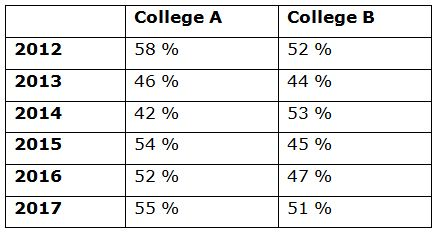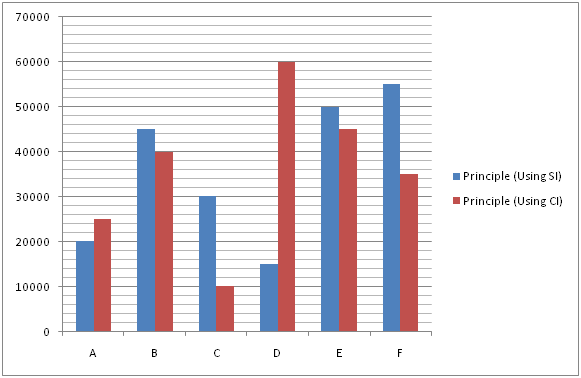# SBI Clerk Mains Quantitative Aptitude Questions 2019 (Day-06)

Dear Aspirants, Our IBPS Guide team is providing a new series of Quantitative Aptitude Questions for SBI Clerk Mains 2019 so the aspirants can practice it on a daily basis. These questions are framed by our skilled experts after understanding your needs thoroughly. Aspirants can practice these new series questions daily to familiarize with the exact exam pattern and make your preparation effective.

Check here for IBPS RRB Clerk Prelims Mock Test 2019

Check here for EPFO Assistant Mock Test 2019

Check here for LIC ADO Mock Test 2019

### Click Here to Subscribe Crack High Level Puzzles & Seating Arrangement Questions PDF 2019 Plan

[WpProQuiz 6784]

Directions (Q. 1 – 5) Study the following information carefully and answer the given questions:

The following table shows the percentage of total number of boys passed in the examination out of the total number of students passed from two different colleges in different years.1) If the total number of girls passed the examination from College A in the year 2012 and College B in the year 2015 is 630 and 660 respectively, then find the ratio between the total number of students passed the examination from College A in the year 2012 to that of total number of students passed the examination from College B in the year 2015?

a) 6 : 7

b) 3 : 2

c) 5 : 4

d) 2 : 1

e) None of these

2) Find the average number of girls passed the examination from college A in the year 2014, 2015, 2016 and 2017 together. The total number of boys passed the examination from college A in the year 2014, 2015, 2016 and 2017 is 3248? (Assume that total number of students passed from College A in all the given years is equal)

a) 788

b) 756

c) 712

d) 694

e) None of these

3) If the total number of boys passed the examination from college B in the year 2013 and 2016 is 528 and 658 respectively, then find the difference between the total number of girls passed the examination from college B in the year 2013  to that in the year 2016?

a) 150

b) 175

c) 125

d) 200

e) None of these

4) If the total number of students passed the examination from college A in the year 2013 is equal to the total number of students passed the examination from college B in the same year, then find the ratio between the total number of girls passed the examination from college A in the year 2013 to that from college B in the same year?

a) 17 : 15

b) 23 : 22

c) 11 : 9

d) 13 : 8

e) None of these

5) The total number of students passed from college A to that of college B in the year 2017 is same, which is equal to 1000. Find the total number of boys passed the examination from college A and B together in the year 2017?

a) 1060

b) 950

c) 1250

d) 1130

e) None of these

Directions (Q. 6 – 10): Study the following information carefully and answer the given questions?

The following bar graph shows the principle amount (In Rs.) invested by six persons on simple interest and compound interest.6) If C invested his amount on C.I for 3 years at 5 % per annum and E invested his amount on S.I at 8 % per annum for 4 years, then find the sum of the compound interest of C and the simple interest of E?

a) Rs. 16528.75

b) Rs. 15242.50

c) Rs. 17576.25

d) Rs. 18527

e) None of these

7) The principle amount invested by A, C and F together in simple interest is approximately what percentage of the principle amount invested by B, D and E together in compound interest?

a) 72 %

b) 85 %

c) 96 %

d) 114 %

e) 60 %

8) Find the difference between the simple interest earned by B after 5 years at 6 % per annum to that of compound interest earned by D after 2 years at 8 % per annum?

a) Rs. 4123

b) Rs. 3516

c) Rs. 2879

d) Rs. 3138

e) None of these

9) The total principle amount invested in simple interest is approximately what percentage of the total principle amount invested in compound interest?

a) 85 %

b) 75 %

c) 50 %

d) 100 %

e) 110 %

10) Find the average simple interest earned by B, C, E and F after 3, 4, 5 and 6 years respectively at 10 % per annum?

a) Rs. 21560

b) Rs. 18525

c) Rs. 20875

d) Rs. 23430

e) None of these

Direction (1-5) :

The total number of girls passed the examination from College A in the year 2012 = 630

(42/100) * Total students passed from College A in the year 2012 = 630

Total students passed from College A = 630 * (100 / 42) = 1500

The total number of girls passed the examination from College B in the year 2015 = 660

(55/100) * Total students passed from College B in the year 2015 = 660

Total students passed from College B in the year 2015

= > 660 * (100 / 55) = 1200

Required ratio = 1500: 1200 = 5: 4

Let the total number of students passed from College A in all the given years be x,

The total number of boys passed the examination from college A in the year 2014, 2015, 2016 and 2017 = 3248

(42 + 54 + 52 + 55) * (x/100) = 3248

x = 3248 * (100/203) = 1600

The average number of girls passed the examination from college A in the year 2014, 2015, 2016 and 2017 together

= > (58 + 46 + 48 + 45) * (1600/100) * (1/4)

= > 788

The total number of boys passed the examination from college B in the year 2013 = 528

(44/100) * Total students passed from college B in 2013 = 528

Total students passed from college B in 2013 = 528 * (100/44) = 1200

The total number of boys passed the examination from college B in the year 2016 = 658

(47/100) * Total students passed from college B in 2016 = 658

Total students passed from college B in 2016 = 658 * (100/47) = 1400

Required difference = 1400 – 1200 = 200

The total number of students passed the examination from college A in the year 2013 = The total number of students passed the examination from college B in the year 2013 = x

Required ratio = [(46/100) * x] : [(44/100) * x]

= > 46 : 44

= > 23 : 22

The total number of students passed from college A in the year 2017 = 1000

The total boys passed from college A in the year 2017

= > 1000 * (55/100) = 550

The total number of students passed from college B in the year 2017 = 1000

The total boys passed from college B in the year 2017

= > 1000 * (51/100) = 510

Required total = 550 + 510 = 1060

The compound interest of C

= > [10000 * (105/100) * (105/100) * (105/100)] – 10000

= > 11576.25 – 10000 = 1576.25

The simple interest of E

= > [50000 * 8 * 4 / 100]

= > 16000

Required sum = 1576.25 + 16000 = Rs. 17576.25

The principle amount invested by A, C and F together in simple interest

= > 20000 + 30000 + 55000 = 105000

The principle amount invested by B, D and E together in compound interest

= > 40000 + 60000 + 45000 = 145000

Required % = (105000 / 145000) * 100 = 72 %

The simple interest earned by B

= > (45000 * 5 * 6) / 100 = Rs. 13500

The compound interest earned by D

= > [60000 * (108/100) * (108/100)] – 60000

= > 69984 – 60000 = Rs. 9984

Required difference = 13500 – 9984 = Rs. 3516

The total principle amount invested in simple interest

= > 20000 + 45000 + 30000 + 15000 + 50000 + 55000 = Rs. 215000

The total principle amount invested in compound interest

= > 25000 + 40000 + 10000 + 60000 + 45000 + 35000 = Rs. 215000

Required % = (215000 / 215000) * 100 = 100 %

The total simple interest earned by B, C, E and F

= > [(45000 * 10 * 3) / 100] + [(30000 * 10 * 4) / 100] + [(50000 * 5 * 10) / 100] + [(55000 * 10 * 6) / 100]

= > 13500 + 12000 + 25000 + 33000 = Rs. 83500

Required average = 83500/4 = Rs. 20875